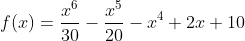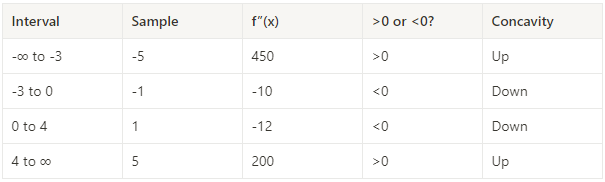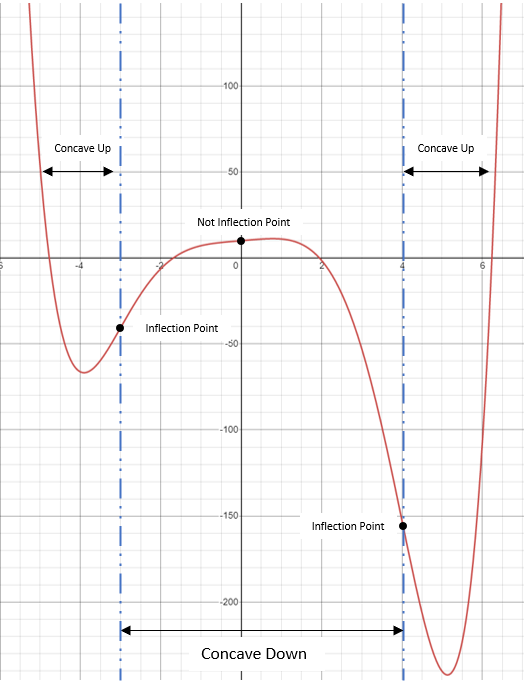## Multi Roots

For the curve represented by the equation below, find the point of inflection(s).Hint
First, find the second derivative of $$f(x)$$ .
Hint 2
Set $$f''(x)=0$$ to solve for the inflection point.
An inflection point is a point on the curve/graph at which concavity changes, and occurs when $$f''(x)=0$$ .
Using the power rule for the first derivative and applying it twice, we’ll get the second derivative power rule:
$$\frac{d^2}{dx^2}[x^n]=\frac{d}{dx}\frac{d}{dx}[x^n]=\frac{d}{dx}[nx^{n-1}]=n\frac{d}{dx}[x^{n-1}]=n(n-1)(x^{n-2})$$$Thus, the second derivative is: $$f''(x)=\frac{(6)(6-1)}{30}x^{6-2}-\frac{(5)(5-1)}{20}x^{5-2}-(4)(4-1)x^{4-2}+(0)+(0)$$$
$$=\frac{(6)(5)}{30}x^{4}-\frac{(5)(4)}{20}x^{3}-(4)(3)x^{2}$$$$$f''(x)=\frac{30}{30}x^{4}-\frac{20}{20}x^{3}-12x^{2}=x^4-x^3-12x^2$$$
To obtain the x-component inflection point(s), solve for $$x$$ when $$f''(x)=0$$ . However, $$f''(x)$$ is a polynomial, so we have to factor to determine its roots:
$$x^4-x^3-12x^2=0$$$$$x^2(x^2-x-12)=0$$$
$$x^2(x+3)(x-4)=0$$$There are three solutions/roots based on the simplified polynomial: $$x=0,-3,\&\:4$$ . However, all three may not be inflection points since we need to determine if concavity changes between these roots. If we choose an arbitrary sample number between the intervals before and after the root points, we can determine concavity direction by plugging in the sample number into $$f''(x)$$ and solving accordingly. If $$f''(x)>0$$ then concavity is up. If $$f''(x)<0$$ , then concavity is down.Therefore, the function is concave up from (-∞, -3), then concave down from (-3, 4), and finally concave up again from (4, ∞), meaning there are only two points of inflection ( $$x=-3\: \&\:4$$ ). The inflection point consists of both a $$x$$ and $$y$$ coordinate. We have solved and confirmed the $$x$$ component, but still need to determine the $$y$$ component. To find $$y$$ , plug the $$x$$ component back into the original function: $$f(-3)=\frac{(-3)^6}{30}-\frac{(-3)^5}{20}-(-3)^4+2(-3)+10$$$
$$=24.3-(-12.15)-81+-6+10=-40.6$$$$$f(4)=\frac{(4)^6}{30}-\frac{(4)^5}{20}-(4)^4+2(4)+10$$$
$$=136.53-51.2-256+8+10=-152.7$$\$
Thus, the inflection points are at (-3, -40.6) and (4, -152.7). Below is the function graphed for visualization:(-3, -40.6) and (4, -152.7)